# Active Power & Reactive power

Let us consider a single phase power circuit in which current lags behind the voltage by an angle φ.
Let the instantaneous electric potential difference v = Vm.sinωt
Then the instantaneous current can be expressed as i = Im. sin(ωt – φ).
Where, Vm and Im are the maximum values of sinusoidally varying electric potential difference and current respectively.
The instantaneous power of the circuit is given by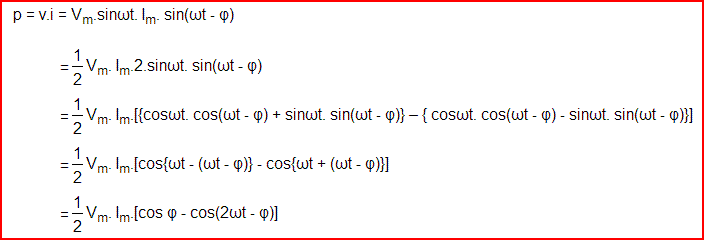## Active Power

### Resistive Power

Let’s take the condition first where the single phase power circuit is fully resistive in nature, that means the phase angle between voltage and current i.e. φ = 0 and hence,From the above equation it is clear that, whatever may be the value of ωt the value of cos2ωt cannot be greater than 1; hence the value of p cannot be negative. The value of p is always positive irrespective of the instantaneous direction of voltage v and current i, that means the energy is flowing in its conventional direction, i.e. from source to load and p is the rate of energy consumption by the load and this is called active power. As this power is consumed due to resistive effect of an electrical circuit, hence sometimes it is also called Resistive Power.

## Reactive Power

### Inductive Power

Now consider a situation when the single phase power circuit is fully inductive, that means the current lags behind the voltage by an angle φ = + 90o. Putting φ = + 90o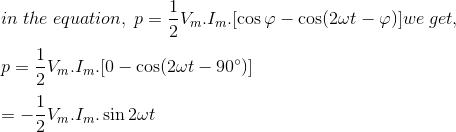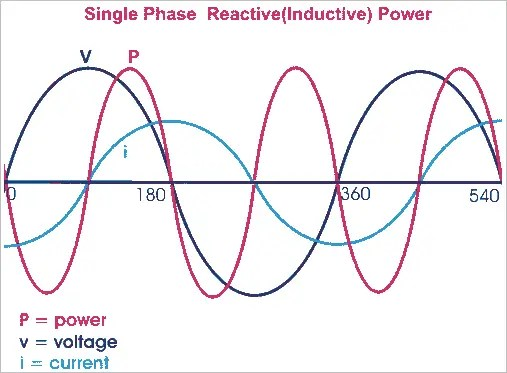In the above expression, it is found that the power is flowing in alternative directions. From 0o to 90o it will have negative half cycle, from 90o to 180o it will have positive half cycle, from 180o to 270o it will have again negative half cycle and from 270o to 360o it will have again positive half cycle. Therefore this power is alternative in nature with a frequency, double of supply frequency. As the power is flowing in alternating direction i.e. from source to load in one half cycle and from load to source in next half cycle, the average value of this power is zero. Therefore this power does not do any useful work. This power is known as reactive power. As the above explained reactive power expression is related to fully inductive circuit, this power is also called inductive power.

This can be concluded as if the circuit is purely inductive, energy will be stored as magnetic field energy during positive half cycle and give away during negative half cycle and rate at which this energy changes, expressed as reactive power of inductor or simply inductive power and this power will have equal positive and negative cycle and the net value will be zero.

### Capacitive Power

Let us now consider the single phase power circuit is fully capacitive, that is the current leads the voltage by 90o, therefore φ = – 90o.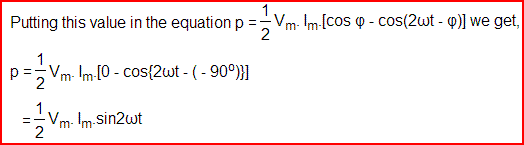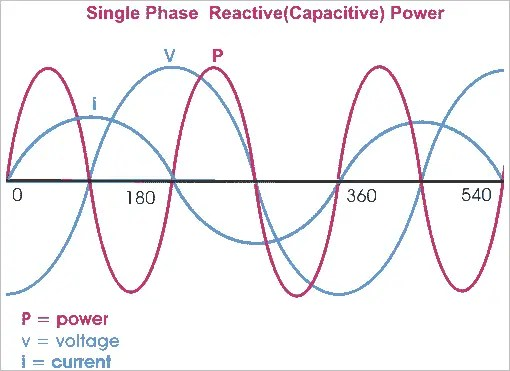Hence in the expression of capacitive power, it is also found that the power is flowing in alternative directions. From 0o to 90o it will have positive half cycle, from 90o to 180o it will have negative half cycle, from 180o to 270o it will have again positive half cycle and from 270o to 360o it will have again negative half cycle. So this power is also alternative in nature with a frequency, double of supply frequency. Therefore, as inductive power, the capacitive power does not do any useful work. This power is also a reactive power.

Reactive power is the form of magnetic energy, flowing per unit time in an electric circuit. Its unit is VAR (Volt Ampere Reactive). This power can never be used in an AC circuit. However, in an electrical DC circuit it can be converted into heat as when a charged capacitor or inductor is connected across a resistor, the energy stored in the element get converted to heat. Our power system operates on AC system and most of the loads used in our daily life, are inductive or capacitive, therefore reactive power is a very important concept from electrical perspective.

The electrical power factor of any equipment determines the amount of reactive power it requires. It is the ratio of real or true power to the total apparent power required by an electrical appliance. These powers can be defined as,Where, θ is the phase difference between voltage and current and cosθ is electrical power factor of the load.Reactive power is always present in a circuit where there is a phase difference between voltage and current in that circuit, such as all our domestic loads are inductive. So, there is a phase difference between voltage and current, and the current lags behind the voltage by certain angle in time domain. An inductive component takes the lagging reactive power and a capacitive component absorbs the leading reactive power, here lagging reactive power refers to magnetic energy and leading reactive power refers to electrostatic energy.

In a typical AC circuit, such as RL circuit (Resistive + Inductive) or RC circuit (Resistive + Capacitive), the reactive power is taken from the supply for a half cycle and returned to the supply for next half cycle. For example power consumed for an RL load is derived as:

V = Vmsinωt , I = Imsin(ωt − θ)Here, Q1sin2ωt is reactive power that’s average value is zero, this shows that reactive power is never utilized.

Referance: electrical4uScroll to Top
Scroll to Top